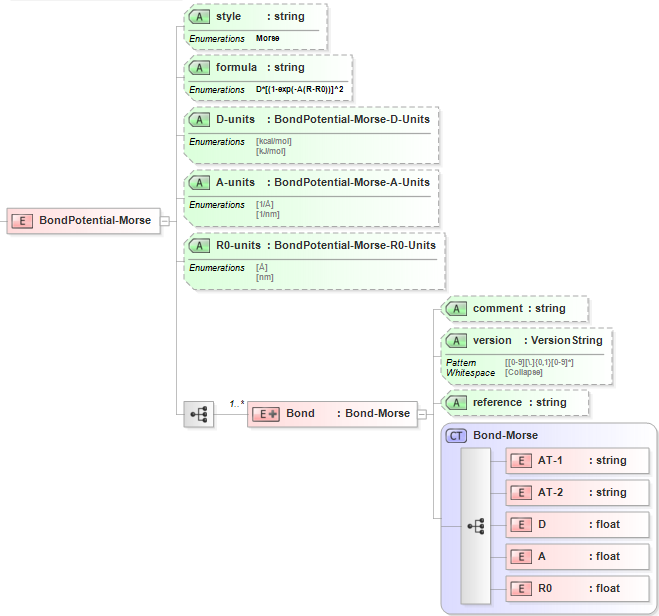3.4. Morse Bond¶

3.4.1. Functional Form¶

The Morse bond potential has the functional form:

$$E=D{{\left[ 1-{{e}^{-\alpha ({{R}_{ij}}-{{R}_{0,ij}})}} \right]}^{2}}$$

The force-field parameters for this potential and units are given by:

 Equation Symbol Parameter Definition Units $$D$$ Depth of the potential well energy $$\alpha$$ Stiffness parameter inverse length $$R_{0,ij}$$ Equilibrium bond length for atoms [i,j] length

3.4.2. XML Schema¶

The XML schema for the Morse bond potential has the following representation (design mode representation using Liquid XML Studio):The relationship between the equation symbols and XML schema notations are given by:

 Parameter Definition Equation Symbol Schema Notation Atom type of atom [i] $$i$$ AT-1 Atom type of atom [j] $$j$$ AT-2 Depth of the potential well $$D$$ D Stiffness parameter $$\alpha$$ A Equilibrium bond length for atoms [i,j] $$R_{0,ij}$$ R0

The general attributes (describing the entire data set) are given by:

 General Attributes Cardinality Value/Definition style Fixed Morse formula Fixed D*[(1-exp(-A(R-R0))]^2 D-units Required Enumerations specified in schema A-units Required Enumerations specified in schema R0-units Required Enumerations specified in schema

The specific attributes (attached to each set of parameters) are given by:

 Specific Attributes Cardinality Value/Definition comment Optional Comment attached to parameter set version Optional Version number of parameter set reference Optional Reference attached to parameter set

Note that an XML document will be rejected from being entered into the WebFF database if a required attribute is left unspecified.